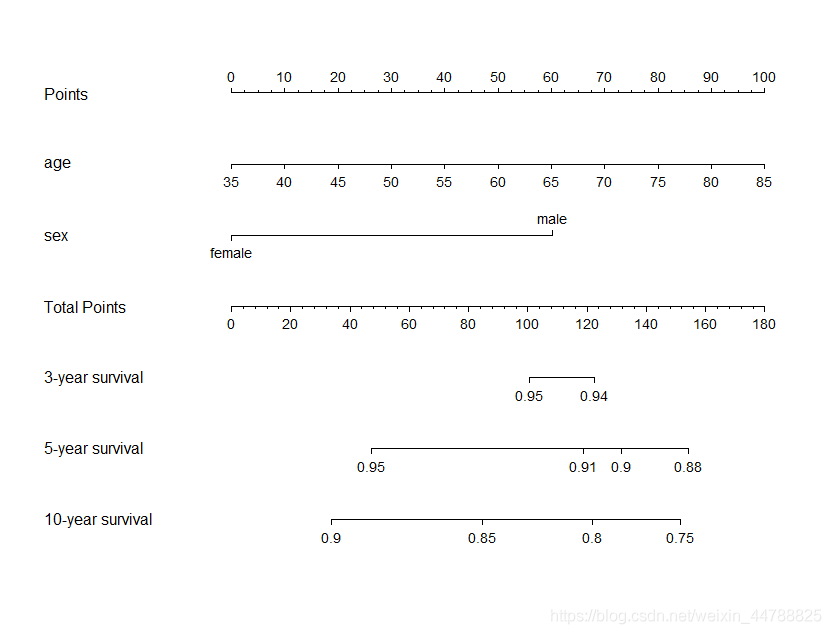# 生存分析定义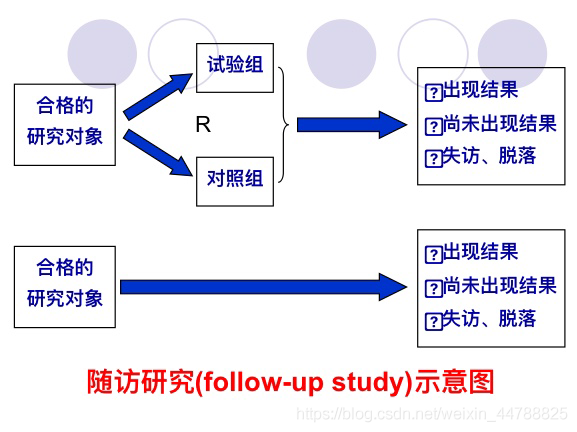# 生存分析的研究内容

1.描述生存过程

2.比较生存过程

3.分析危险因素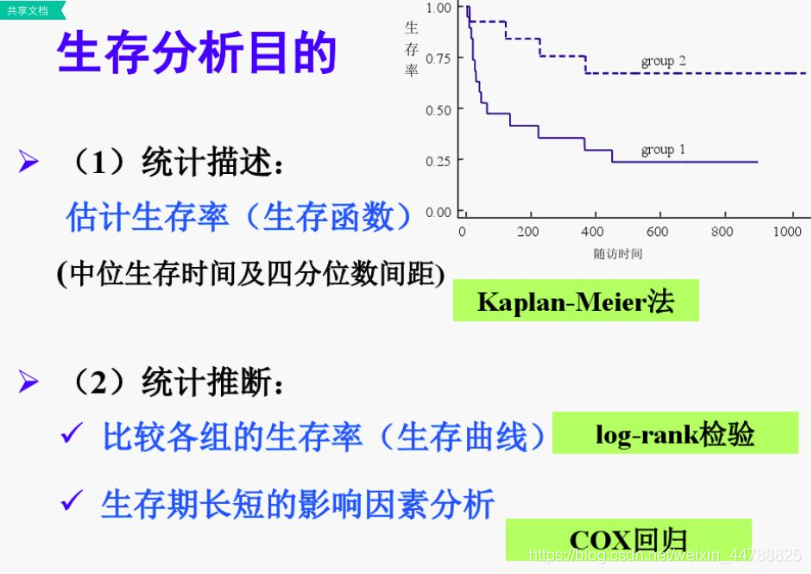# 生存分析资料的特点

1.样本由随机抽样方法获得，要有一定的数量，死亡例数和比例不能太少
2.完整数据所占的比例不能太少，即截尾值不宜太多，一般截尾少于20%
3.截尾值出现的原因无偏性，为防止偏性常常对被截尾的研究对象的年龄、职业、地区、病情轻重等情况进行分析
4.生存时间尽可能精确
5.缺项要尽量补齐

1.蕴含有结局和时间两个方面的信息
2.结局为两分类互斥事件
3一般是通过随访收集得到，随访观察往往是从某统一时间点(如入院或实施手术等某种处理措施后)开始，观察到某规定时间点截止。
4.常因失访等原因造成研究对象的生存时间数据不完整，分布类型复杂，不能简中地套用以前的多因素分析方法

# 生存资料的基本名词概念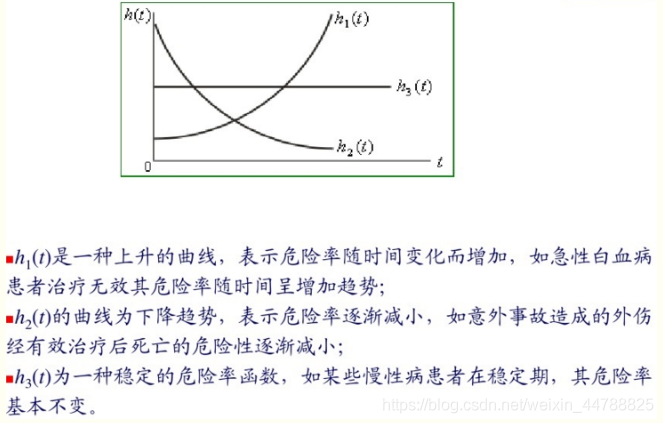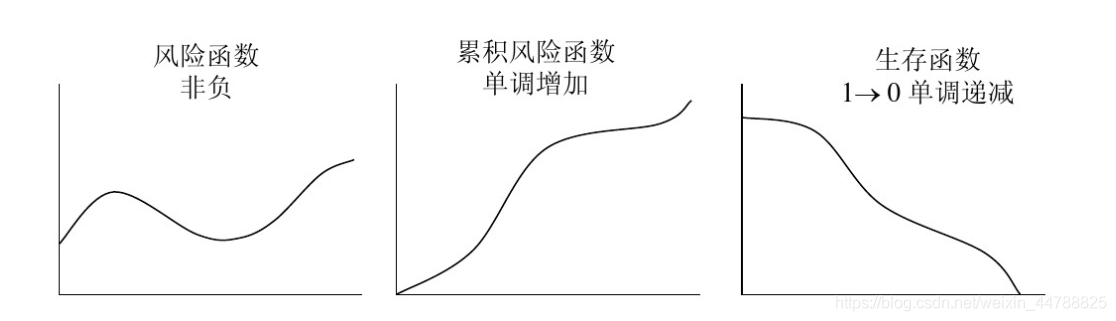# R语言+生存分析

## 一、生存过程描述

K-M曲线的绘制：

``````library(survival)
library(survminer)

#载入数据
> data("lung")
inst time status age sex ph.ecog ph.karno pat.karno meal.cal wt.loss
1    3  306      2  74   1       1       90       100     1175      NA
2    3  455      2  68   1       0       90        90     1225      15
3    3 1010      1  56   1       0       90        90       NA      15
4    5  210      2  57   1       1       90        60     1150      11
5    1  883      2  60   1       0      100        90       NA       0
6   12 1022      1  74   1       1       50        80      513       0
``````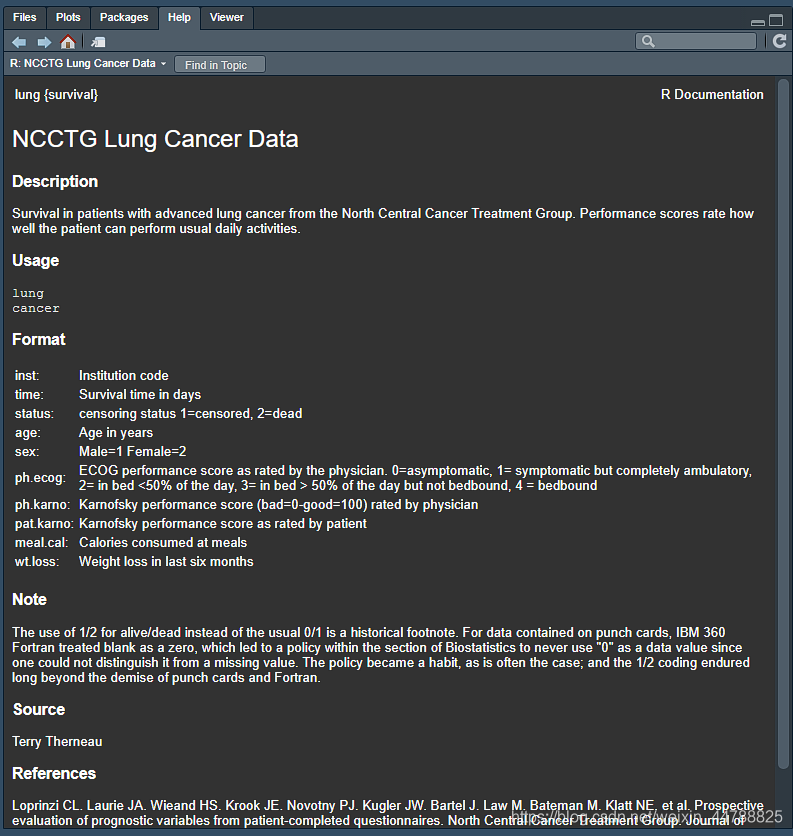``````Surv(lung\$time,lung\$status)
?Surv
``````

``````> Surv(lung\$time,lung\$status)
  306   455  1010+  210   883  1022+  310   361   218   166   170
  654   728    71   567   144   613   707    61    88   301    81
  624   371   394   520   574   118   390    12   473    26   533
  107    53   122   814   965+   93   731   460   153   433   145
  583    95   303   519   643   765   735   189    53   246   689
   65     5   132   687   345   444   223   175    60   163    65
  208   821+  428   230   840+  305    11   132   226   426   705
……
``````

``````fit <- survfit(Surv(time, status) ~ sex, data = lung)
?survfit
#survfit.formula(Surv(time, status) ~ sex, data = lung)
``````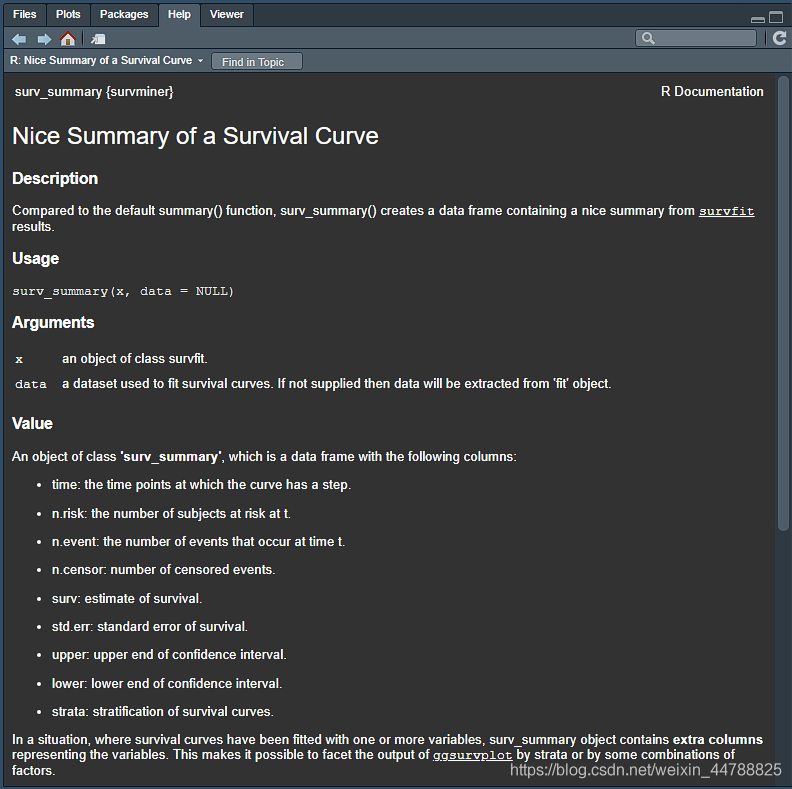``````library(survminer)
ggsurvplot(fit,
pval = TRUE, conf.int = TRUE,#可信区间带
#cumevents=T,
risk.table = TRUE, # 添加 risk table
risk.table.col = "strata", # 改变这个表的颜色，一般就根据曲线的分组来
linetype = "strata", # 改变线条的形状，一般就分组了
surv.median.line = "hv", # 中位生存时间标识
ggtheme = theme_bw(), # 看帮助文档，里面有很多中主题
palette = c("#E7B800", "#2E9FDF"),
#fun = "event" #用来做累积
)
?ggsurvplot
``````

## 二、生存过程比较

``````surv_diff <- survdiff(Surv(time, status) ~ sex, data = lung)
?survdiff
``````

## 三、分析危险因素

Cox比例风险模型的因变量由h(t)表示的危险函数表示。简而言之，危险函数可以解释为在时间t死亡的风险。可以估计如下：
h(t) = h0(t) * exp(b1X1 + b2X2 + …… + bpXp)

①当协变量Xp为二分类变量0，1时，按公式，其对应的RR与回归系数的关系RR = exp(bp)，其流行病学含义为，与赋值为0的个体相比，赋值为1的个体的死亡风险增加RR-1倍
②当协变量为连续型变量时，用Xp1和Xp2表示不同情况下的取值，则RR = exp[bp(Xp1 - Xp2)]
③当协变量为多分类变量时，则可以设置哑变量，以其中一份分类为参比，估计其他分类的相对危险度。
RR > 1（即：b> 0）的协变量被称为不良预后因素
RR < 1（即：b <0）的协变量被称为良好的预后因子

``````res.cox <- coxph(Surv(time,status)~sex + age + ph.ecog + ph.karno + pat.karno + meal.cal + wt.loss,
data=lung)
``````

``````ggforest(res.cox,main="hazard ratio",
cpositions=c(0.02,0.22,0.4),
fontsize=0.8,refLabel="reference",noDigits=2)
``````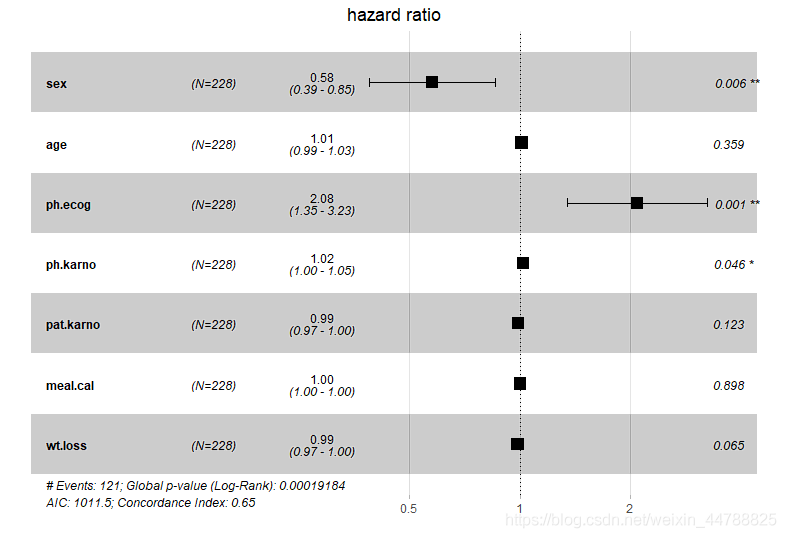``````colon <- within(lung, {
sex <- factor(sex, labels = c("female", "male"))
ph.ecog <- factor(ph.ecog, labels = c("asymptomatic", "symptomatic",'in bed <50%','in bed >50%'))
})

res.cox <- coxph(Surv(time,status)~sex + age + ph.ecog + ph.karno + pat.karno + meal.cal + wt.loss,
data=colon)

ggforest(res.cox,main="hazard ratio",
cpositions=c(0.02,0.22,0.4),
fontsize=0.8,refLabel="reference",noDigits=2)
``````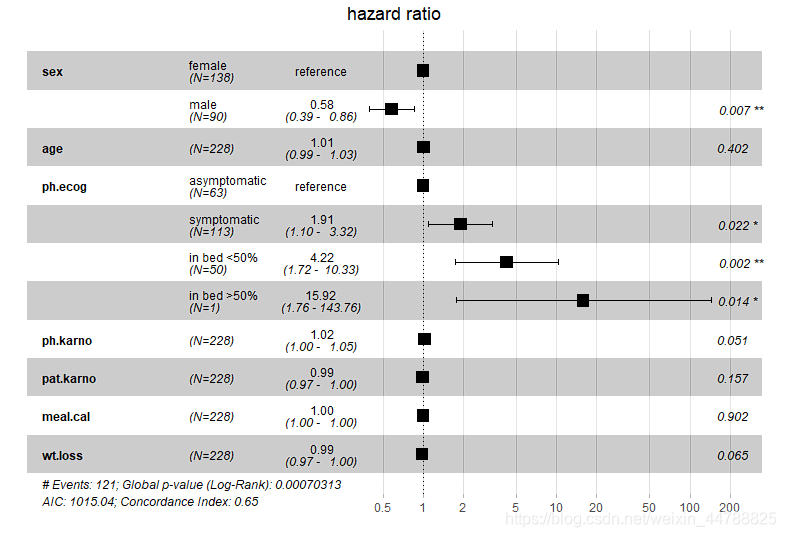# 补充：列线图

``````library(Hmisc); library(grid); library(lattice);library(Formula); library(ggplot2)
library(rms)
library(survival)
``````

``````data("lung")

lung\$sex <-
factor(lung\$sex,
levels = c(1,2),
labels = c("male", "female"))## 添加变量标签以便后续说明

``````

``````## 绘制logisitc回归的风险预测值的nomogram图
f1 <- lrm(status~ age + sex, data = lung) ## 构建logisitc回归模型
?nomogram
nom <- nomogram(f1,  fun= function(x)1/(1+exp(-x)),# or fun=plogis这个函数是用来建立要预测目标
lp=T, funlabel="Risk")

plot(nom)
``````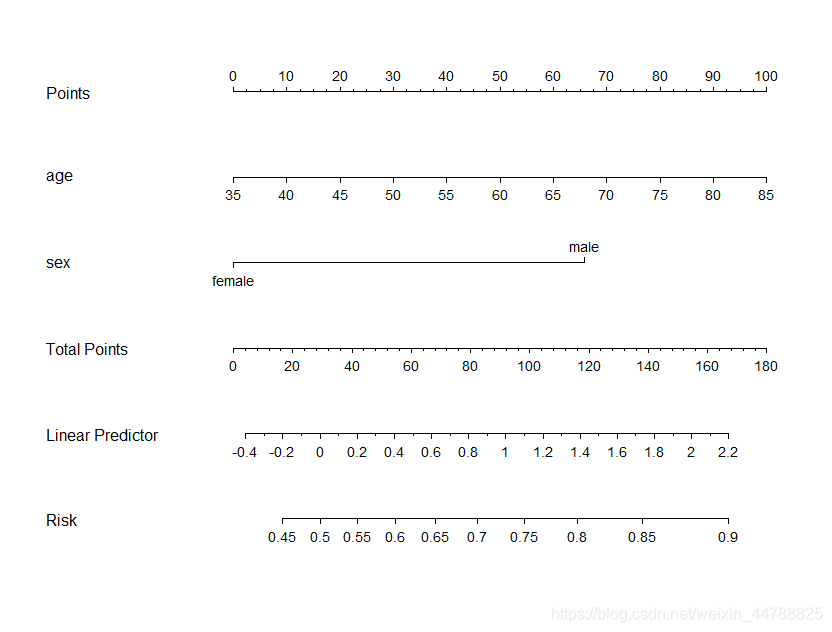``````## 绘制Cox比例风险模型预测的nomogram图
f <- cph(Surv(time, status) ~ age + sex , x=T, y=T, surv=T,time.inc=36, data=lung) # time.inc参数，与列线图中预测的几年几年生存率有关

surv <- Survival(f) # 构建生存预测函数，surv就是我们自定义的一个函数

nom <- nomogram(f, fun=list(function(x) surv(36, x),  # 36代表3*12，就是三年生存率
function(x) surv(60, x),
function(x) surv(120, x)),
lp=F,
funlabel=c("3-year survival", "5-year survival", "10-year survival"),
maxscale=100, # 线段上的刻度最大值，倒不是很重要，都是等比例的
fun.at=list(c(0.95, 0.94), # 与fun对应的，是生存率线段的刻度
c(0.97,0.95, 0.91, 0.9, 0.88),
c(0.95, 0.9, 0.85, 0.8, 0.75)))

plot(nom)
``````